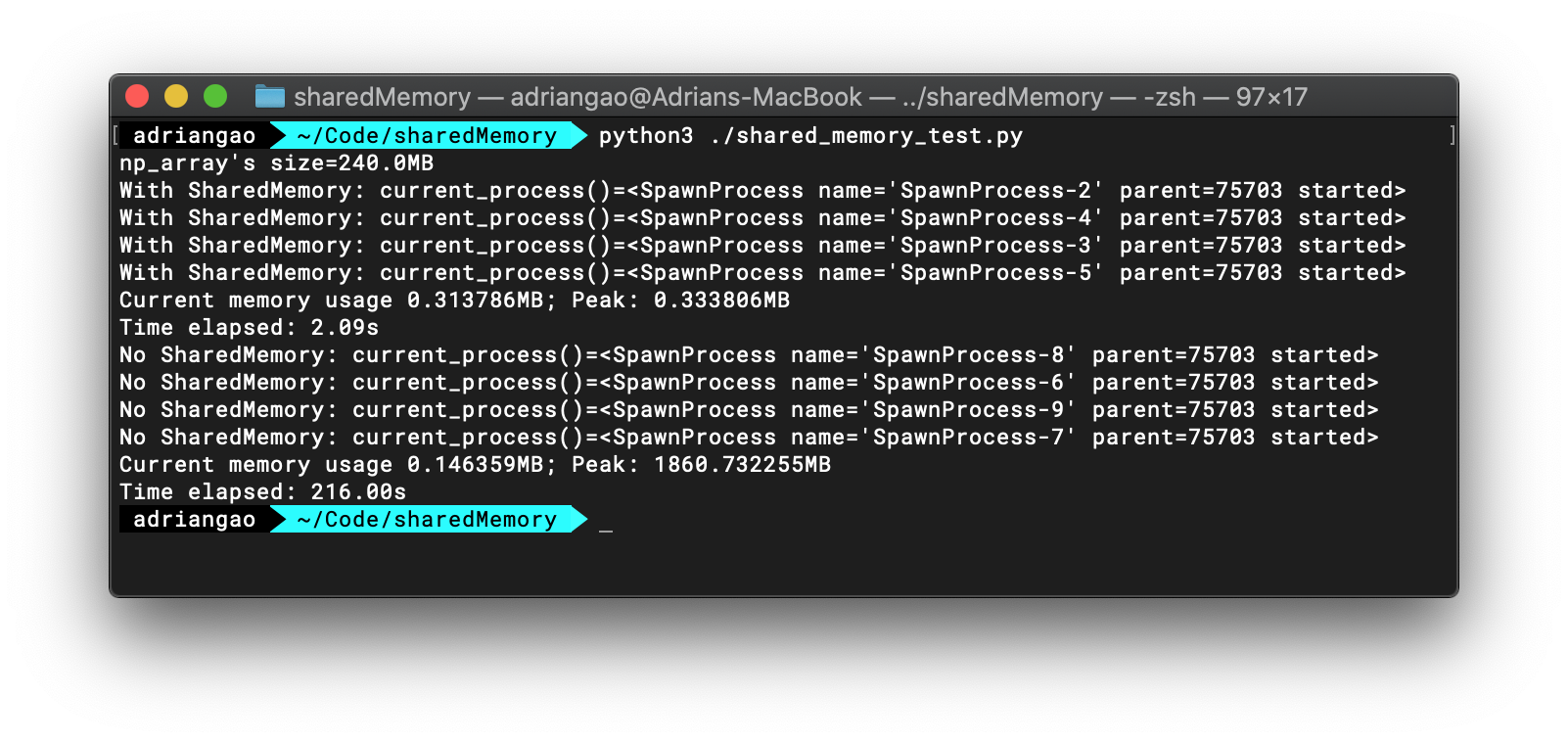# Python Shared Memory in Multiprocessing¶

Python 3.8 introduced a new module multiprocessing.shared_memory that provides shared memory for direct access across processes. My test shows that it significantly reduces the memory usage, which also speeds up the program by reducing the costs of copying and moving things around.1

## Test¶

In this test, I generated a 240MB numpy.recarray from a pandas.DataFrame with datetime, int and str typed columns. I used numpy.recarray because it can preserve the dtype of each column, so that later I can reconstruct the same array from the buffer of shared memory.

I performed a simple numpy.nansum on the numeric column of the data using two methods. The first method uses multiprocessing.shared_memory where the 4 spawned processes directly access the data in the shared memory. The second method passes the data to the spawned processes, which effectively means each process will have a separate copy of the data.

### Test Result¶A quick run of the test code below shows that the first method based on shared_memory uses minimal memory (peak usage is 0.33MB) and is much faster (2.09s) than the second one where the entire data is copied and passed into each process (peak memory usage of 1.8G and takes 216s). More importantly, the memory usage under the second method is consistently high.

### Test Code¶

  1 2 3 4 5 6 7 8 9 10 11 12 13 14 15 16 17 18 19 20 21 22 23 24 25 26 27 28 29 30 31 32 33 34 35 36 37 38 39 40 41 42 43 44 45 46 47 48 49 50 51 52 53 54 55 56 57 58 59 60 61 62 63 64 65 66 67 68 69 70 71 72 73 74 75 from multiprocessing.shared_memory import SharedMemory from multiprocessing.managers import SharedMemoryManager from concurrent.futures import ProcessPoolExecutor, as_completed from multiprocessing import current_process, cpu_count, Process from datetime import datetime import numpy as np import pandas as pd import tracemalloc import time def work_with_shared_memory(shm_name, shape, dtype): print(f'With SharedMemory: {current_process()=}') # Locate the shared memory by its name shm = SharedMemory(shm_name) # Create the np.recarray from the buffer of the shared memory np_array = np.recarray(shape=shape, dtype=dtype, buf=shm.buf) return np.nansum(np_array.val) def work_no_shared_memory(np_array: np.recarray): print(f'No SharedMemory: {current_process()=}') # Without shared memory, the np_array is copied into the child process return np.nansum(np_array.val) if __name__ == "__main__": # Make a large data frame with date, float and character columns a = [ (datetime.today(), 1, 'string'), (datetime.today(), np.nan, 'abc'), ] * 5000000 df = pd.DataFrame(a, columns=['date', 'val', 'character_col']) # Convert into numpy recarray to preserve the dtypes (1) np_array = df.to_records(index=False, column_dtypes={'character_col': 'S6'}) del df shape, dtype = np_array.shape, np_array.dtype print(f"np_array's size={np_array.nbytes/1e6}MB") # With shared memory # Start tracking memory usage tracemalloc.start() start_time = time.time() with SharedMemoryManager() as smm: # Create a shared memory of size np_arry.nbytes shm = smm.SharedMemory(np_array.nbytes) # Create a np.recarray using the buffer of shm shm_np_array = np.recarray(shape=shape, dtype=dtype, buf=shm.buf) # Copy the data into the shared memory np.copyto(shm_np_array, np_array) # Spawn some processes to do some work with ProcessPoolExecutor(cpu_count()) as exe: fs = [exe.submit(work_with_shared_memory, shm.name, shape, dtype) for _ in range(cpu_count())] for _ in as_completed(fs): pass # Check memory usage current, peak = tracemalloc.get_traced_memory() print(f"Current memory usage {current/1e6}MB; Peak: {peak/1e6}MB") print(f'Time elapsed: {time.time()-start_time:.2f}s') tracemalloc.stop() # Without shared memory tracemalloc.start() start_time = time.time() with ProcessPoolExecutor(cpu_count()) as exe: fs = [exe.submit(work_no_shared_memory, np_array) for _ in range(cpu_count())] for _ in as_completed(fs): pass # Check memory usage current, peak = tracemalloc.get_traced_memory() print(f"Current memory usage {current/1e6}MB; Peak: {peak/1e6}MB") print(f'Time elapsed: {time.time()-start_time:.2f}s') tracemalloc.stop() 
1. Check the note below for preventing segfault.

## Important Note¶

Warning

A very important note about using multiprocessing.shared_memory, as at June 2020, is that the numpy.ndarray cannot have a dtype=dtype('O'). That is, the dtype cannot be dtype(object). If it is, there will be a segmentation fault when child processes try to access the shared memory and dereference it. It happens when the column contains strings.

To solve this problem, you need to specify the dtype in df.to_records(). For example:

np_array = df.to_records(index=False，column_dtypes={'character_col': 'S6'})


Here, we specify that character_col contains strings of length 6. If it contains Unicode, we can use 'U6' instead. Longer strings will then be truncated at the specified length. As such, there won't be anymore segfault.

1. This test is performed on a 2017 12-inch MacBook with 1.3 GHz Dual-Core Intel Core i5 and 8 GB 1867 MHz LPDDR3 RAM.